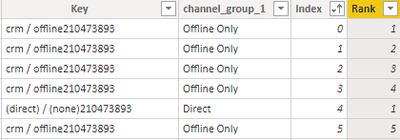cancel
Showing results for
Did you mean:Frequent Visitor

## Getting Rank of Text Column which has duplicate records

I am not sure how to mention this. I have a simple table as below. I am trying to get the rank basis on 'Name' Column which has duplicate record. Can any one help whether this can be achieved in power bi? Thanks in Advance.

 Name Desired Output (Rank) A 1 A 2 A 3 A 4 B 1 B 2 B 3 C 1 C 2 C 3 C 4 C 5 C 6

2 ACCEPTED SOLUTIONSSolution Sage

Once you've added your index column, you can use the DAX expression below to create your rank column:

``````Rank =
VAR RowName = Table1[Name]
VAR Result =
RANKX(
FILTER(
ALL( Table1 ),
Table1[Name] = RowName
),
Table1[Index],
,ASC
)
RETURN Result``````

Best regards,
MartynAnonymous
Not applicable

Create one index column and then create one more column as below.

Rank = CALCULATE(COUNT('Table'[Col]),FILTER('Table','Table'[Col]=EARLIER('Table'[Col]) && 'Table'[Index]<=EARLIER('Table'[Index])))

Thanks & regards,
Pravin Wattamwar

If I resolve your problem Mark it as a solution and give kudos.
7 REPLIES 7Helper I

I have a similar problem with ranking. What I need is to have all channel_grouping_1 have the same rank. Offline Only 1 across all lines, Direct 2 across all lines etc. Can anyone help?Many thanksCommunity Champion

the easiest way is to add a Custom Index Column in Power Query Editor mode

then try to create a calculated DAX column like

``````RANK =
var _RowsBefore = COUNTROWS(FILTER('Table', 'Table'[Name]<EARLIER('Table'[Name])))
RETURN
rankx(FILTER('Table', 'Table'[Name]=EARLIER('Table'[Name])), 'Table'[Index],,ASC) + _RowsBefore``````

do not hesitate to give a kudo to useful posts and mark solutions as solutionFrequent Visitor

HiSolution Sage

Once you've added your index column, you can use the DAX expression below to create your rank column:

``````Rank =
VAR RowName = Table1[Name]
VAR Result =
RANKX(
FILTER(
ALL( Table1 ),
Table1[Name] = RowName
),
Table1[Index],
,ASC
)
RETURN Result``````

Best regards,
MartynFrequent Visitor

Regards!Anonymous
Not applicable

Create one index column and then create one more column as below.

Rank = CALCULATE(COUNT('Table'[Col]),FILTER('Table','Table'[Col]=EARLIER('Table'[Col]) && 'Table'[Index]<=EARLIER('Table'[Index])))

Thanks & regards,
Pravin Wattamwar

If I resolve your problem Mark it as a solution and give kudos.Frequent Visitor

Hi @Anonymous

Perfect, This is also a solution to my concern. Thanks a lot for your help.

Regards,